# 机器学习| 朴素贝叶斯详解

## 朴素贝叶斯基础

### 基本概念

#### 条件概率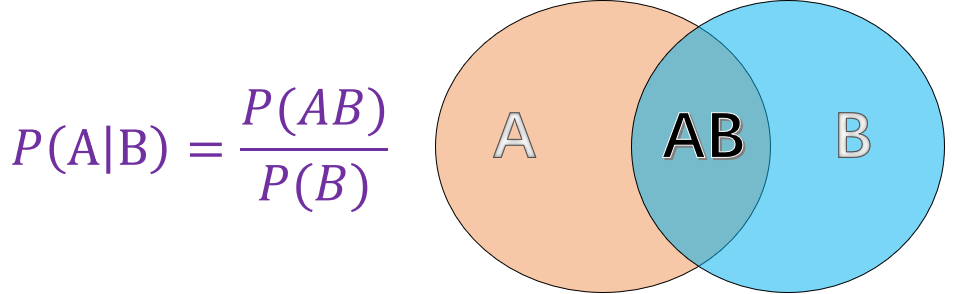• $P(A)$ 表示 $A$ 事件发生的概率。
• $P(B)$ 表示 $B$ 事件发生的概率。
• $P(AB)$ 表示 $A, B$ 事件同时发生的概率。

#### 贝叶斯定理

$$P(B \mid A)=\frac{P(AB)}{P(A)} \tag1$$

$$P(AB)=P(A \mid B)*P(B) \tag2$$

$$P(B \mid A)=\frac{P(AB)}{P(A)}=\frac{P(A \mid B)*P(B)}{P(A)} \tag{3}$$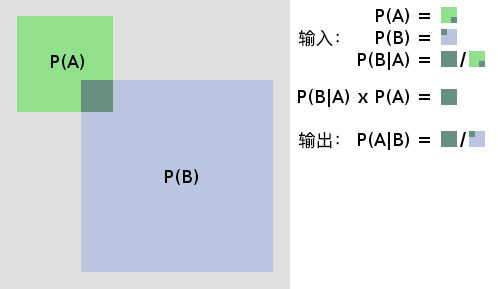### 什么是朴素贝叶斯

$$P(B \mid A)=\frac{P(A \mid B)*P(B)}{P(A)} \tag{4}$$

$$P(类别 \mid 特征)=\frac{P(特征 \mid 类别) * P(类别)}{P(特征)} \tag{5}$$

## 朴素贝叶斯算法实现

$$X = \left { a_{1},a_{2},a_{3},…,a_{n} \right }$$

$$Y = \left {y_{1},y_{2},y_{3},…,y_{m} \right }$$

### 生成示例数据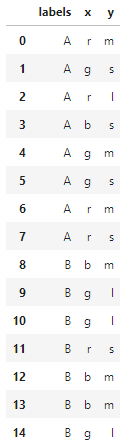### 参数估计

#### 极大似然估计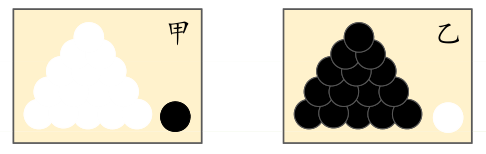$$P(y_{i}=c_{k})=\frac{\sum_{N}^{i=1}I(y_{i}=c_{k})}{N},k=1,2,3,…,m \tag{6}$$

$P(特征 \mid 种类)$ 由于公式较为繁琐这里先不给出，直接用叙述的方式能更清晰地帮助理解：

#### 贝叶斯估计

$$P(y_{i}=c_{k})=\frac{\sum_{N}^{i=1}I(y_{i}=c_{k})+\lambda }{N+k\lambda} \tag{8}$$

### 朴素贝叶斯的三种常见模型

#### 伯努利模型

• 当特征值 $x_{i}=1$ 时，$P(x_{i} \mid y_{k})=P(x_{i}=1 \mid y_{k})$;
• 当特征值 $x_{i}=0$ 时，$P(x_{i} \mid y_{k})=P(x_{i}=0 \mid y_{k})$。

#### 高斯模型

$$P(x_{i}|y_{k})=\frac{1}{\sqrt{2\pi}\sigma_{y_{k},i}}exp(-\frac{(x-\mu_{y_{k},i}) ^{2}}{2\sigma ^{2}{y{k}},i})$$

• $\mu_{y_{k},i}$ 表示类别为 $y_{k}$ 的样本中，第 $i$ 维特征的均值。
• $\sigma ^{2}{y{k}},i$ 表示类别为 $y_{k}$ 的样本中，第 $i$ 维特征的方差。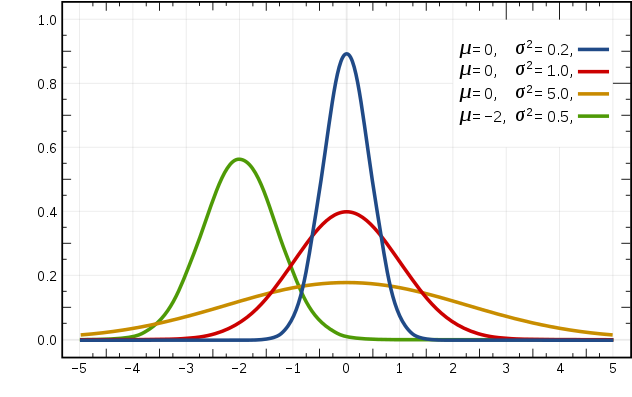laugh12321

2019-01-19

2020-10-23